Anúncio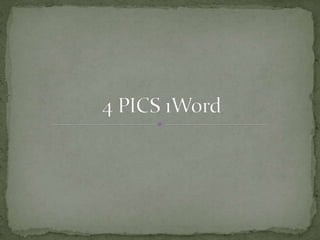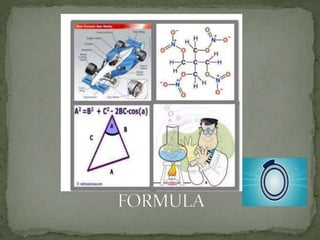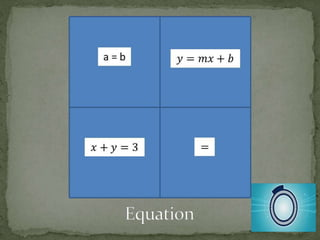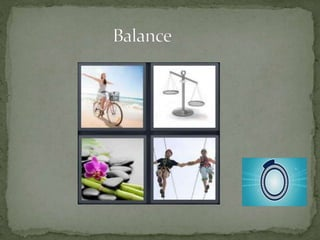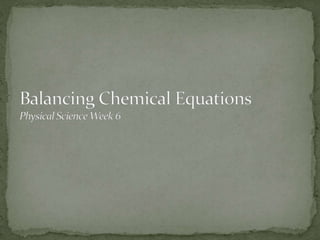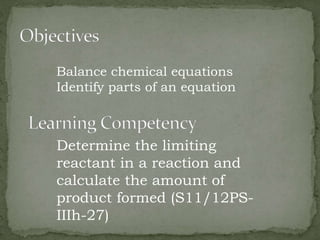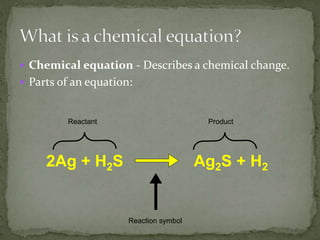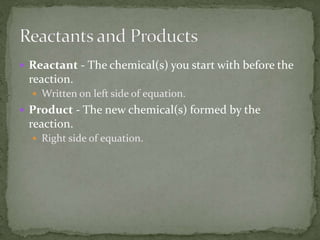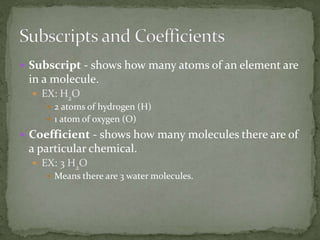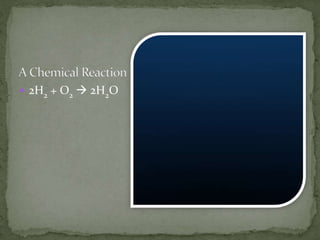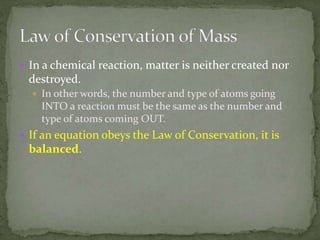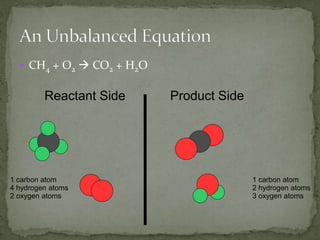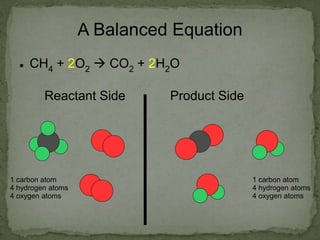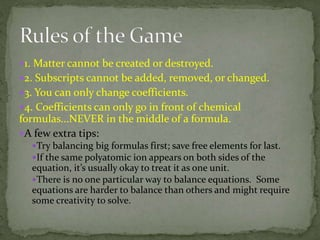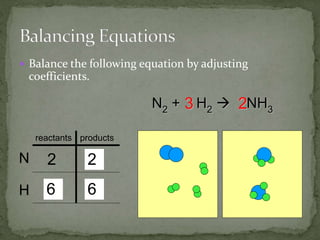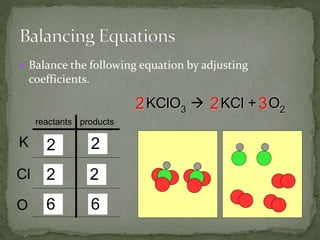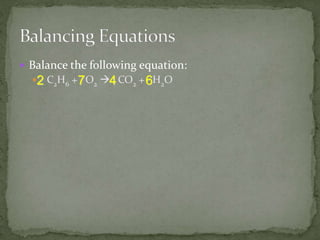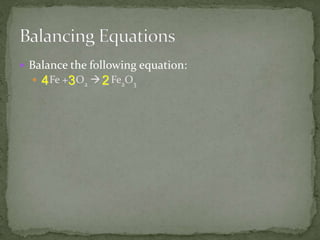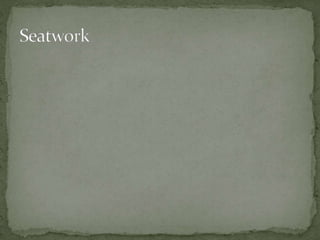1 de 19
Anúncio

### Balancing_Chemical_Equations.pptx

1. Balance chemical equations Identify parts of an equation Determine the limiting reactant in a reaction and calculate the amount of product formed (S11/12PS- IIIh-27)
2.  Chemical equation - Describes a chemical change.  Parts of an equation: 2Ag + H2S Ag2S + H2 Reactant Product Reaction symbol
3.  Reactant - The chemical(s) you start with before the reaction.  Written on left side of equation.  Product - The new chemical(s) formed by the reaction.  Right side of equation.
4.  Subscript - shows how many atoms of an element are in a molecule.  EX: H2O  2 atoms of hydrogen (H)  1 atom of oxygen (O)  Coefficient - shows how many molecules there are of a particular chemical.  EX: 3 H2O  Means there are 3 water molecules.
5.  2H2 + O2  2H2O
6.  In a chemical reaction, matter is neither created nor destroyed.  In other words, the number and type of atoms going INTO a reaction must be the same as the number and type of atoms coming OUT.  If an equation obeys the Law of Conservation, it is balanced.
7.  CH4 + O2  CO2 + H2O Reactant Side Product Side 1 carbon atom 4 hydrogen atoms 2 oxygen atoms 1 carbon atom 2 hydrogen atoms 3 oxygen atoms
8. A Balanced Equation  CH4 + 2O2  CO2 + 2H2O Reactant Side Product Side 1 carbon atom 4 hydrogen atoms 4 oxygen atoms 1 carbon atom 4 hydrogen atoms 4 oxygen atoms
9. 1. Matter cannot be created or destroyed. 2. Subscripts cannot be added, removed, or changed. 3. You can only change coefficients. 4. Coefficients can only go in front of chemical formulas...NEVER in the middle of a formula. A few extra tips: Try balancing big formulas first; save free elements for last. If the same polyatomic ion appears on both sides of the equation, it’s usually okay to treat it as one unit. There is no one particular way to balance equations. Some equations are harder to balance than others and might require some creativity to solve.
10.  Balance the following equation by adjusting coefficients. reactants products N H N2 + H2  NH3 2 2 1 3 2 2 6 3 6
11.  Balance the following equation by adjusting coefficients. KClO3  KCl + O2 reactants products K Cl O 1 1 1 1 3 2 3 2 2 2 6 6 2 2 2
12.  Balance the following equation:  C2H6 + O2  CO2 + H2O 4 6 7 2
13.  Balance the following equation:  Fe + O2  Fe2O3 2 3 4
Anúncio